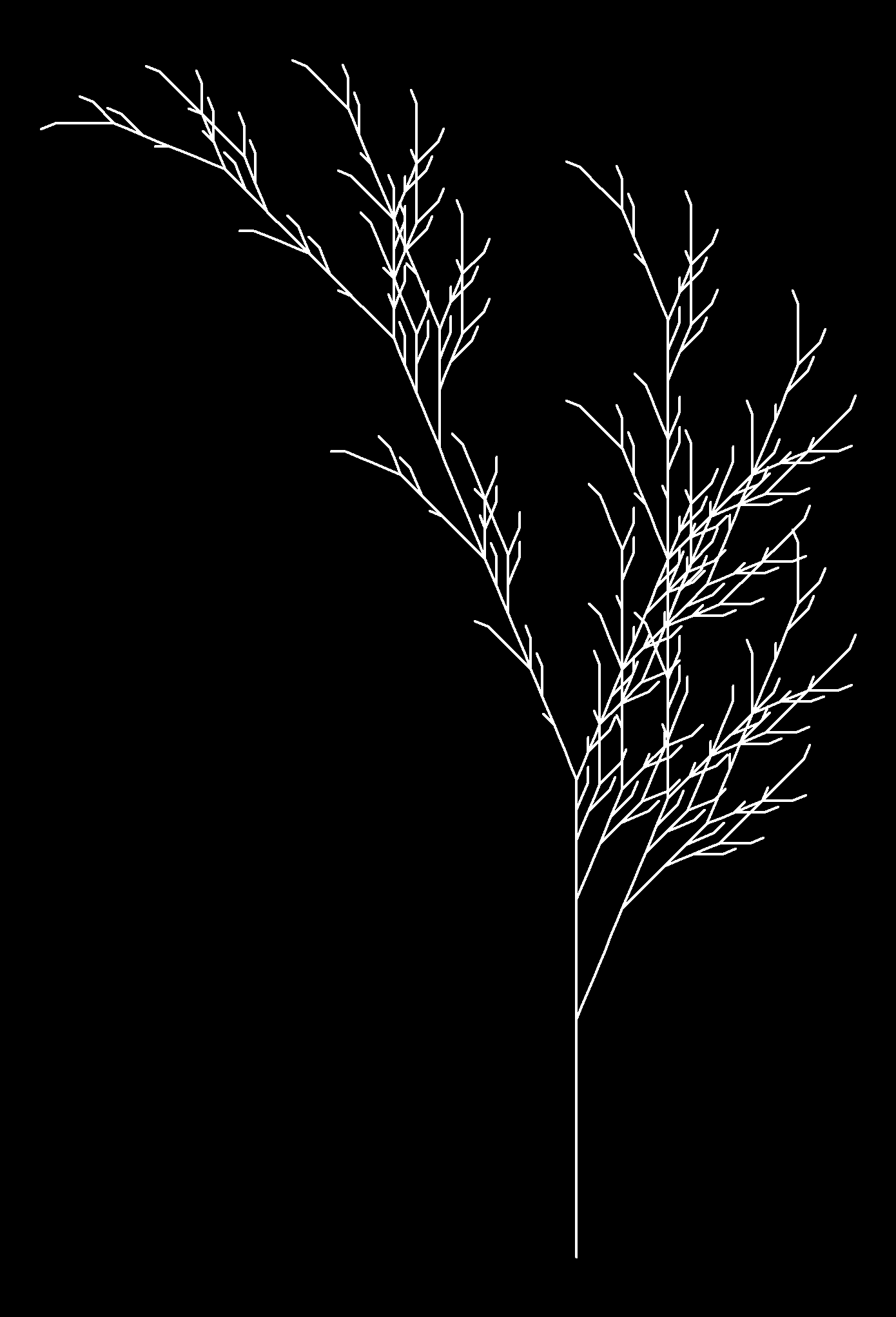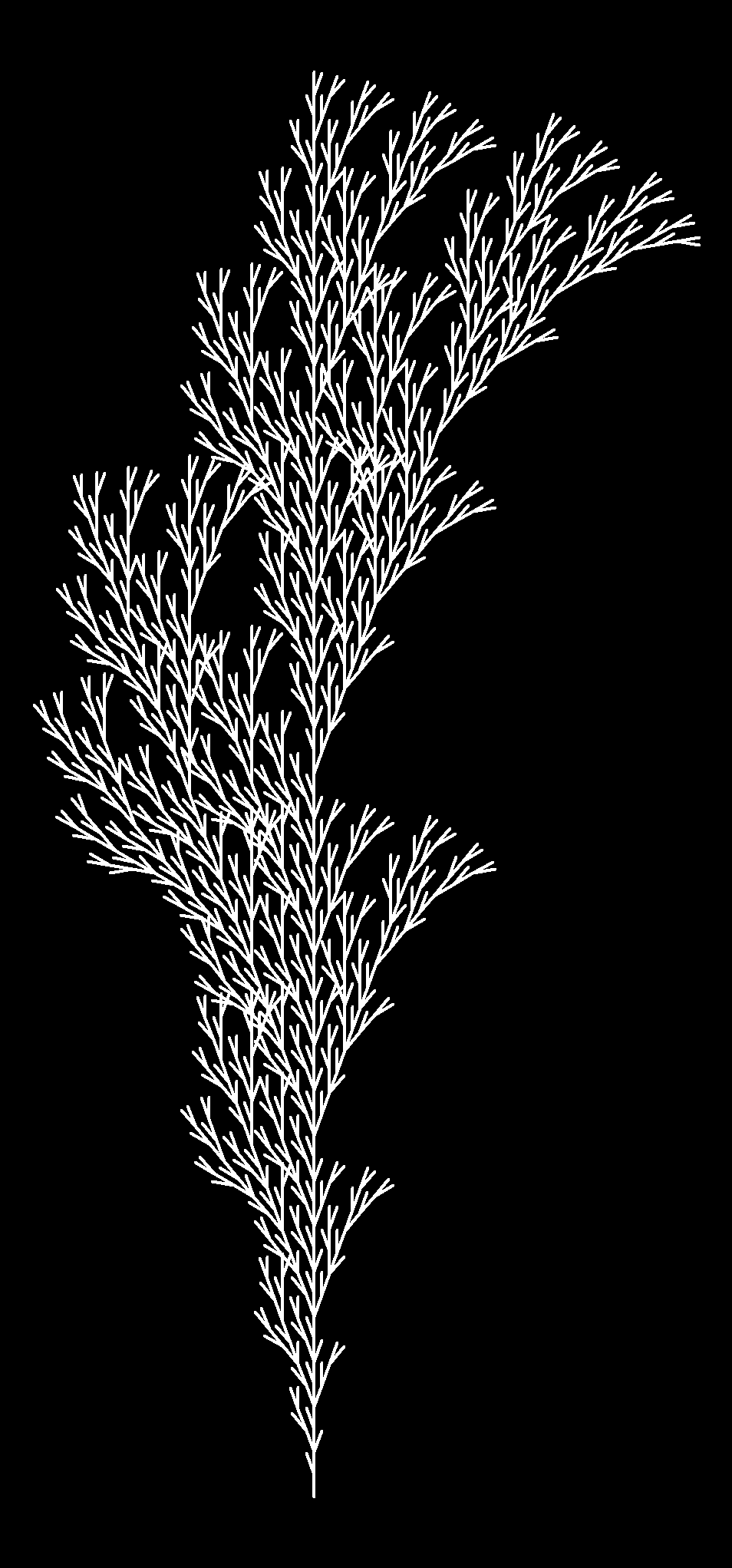# Plants

Blue dragonflies dart to and fro
I tie my life to your balloon and let it go
(Warm Foothills, Alt-J)

In my last post I did some drawings based on L-Systems. These drawings are done sequentially. At any step, the state of the drawing can be described by the position (coordinates) and the orientation of the pencil. In that case I only used two kind of operators: drawing a straight line and turning a constant angle. Today I used two more symbols to do stack operations:

• “[“ Push the current state (position and orientation) of the pencil onto a pushdown
operations stack
• “]” Pop a state from the stack and make it the current state of the pencil (no line is drawn)

These operators allow to return to a previous state to continue drawing from there. Using them you can draw plants like these:Each image corresponds to a different axiom, rules, angle and depth. I described these terms in my previous post. If you want to reproduce them you can find the code below (each image corresponds to a different set of axiom, rules, angle and depth parameters). Change colors, add noise to angles, try your own plants … I am sure you will find nice images:

```
library(gsubfn)
library(stringr)
library(dplyr)
library(ggplot2)

#Plant 1
axiom="F"
rules=list("F"="FF-[-F+F+F]+[+F-F-F]")
angle=22.5
depth=4

#Plant 2
axiom="X"
rules=list("X"="F[+X][-X]FX", "F"="FF")
angle=25.7
depth=7

#Plant 3
axiom="X"
rules=list("X"="F[+X]F[-X]+X", "F"="FF")
angle=20
depth=7

#Plant 4
axiom="X"
rules=list("X"="F-[[X]+X]+F[+FX]-X", "F"="FF")
angle=22.5
depth=5

#Plant 5
axiom="F"
rules=list("F"="F[+F]F[-F]F")
angle=25.7
depth=5

#Plant 6
axiom="F"
rules=list("F"="F[+F]F[-F][F]")
angle=20
depth=5

for (i in 1:depth) axiom=gsubfn(".", rules, axiom)

actions=str_extract_all(axiom, "\\d*\\+|\\d*\\-|F|L|R|\\[|\\]|\\|") %>% unlist

status=data.frame(x=numeric(0), y=numeric(0), alfa=numeric(0))
points=data.frame(x1 = 0, y1 = 0, x2 = NA, y2 = NA, alfa=90, depth=1)

for (action in actions)
{
if (action=="F")
{
x=points[1, "x1"]+cos(points[1, "alfa"]*(pi/180))
y=points[1, "y1"]+sin(points[1, "alfa"]*(pi/180))
points[1,"x2"]=x
points[1,"y2"]=y
data.frame(x1 = x, y1 = y, x2 = NA, y2 = NA,
alfa=points[1, "alfa"],
depth=points[1,"depth"]) %>% rbind(points)->points
}
if (action %in% c("+", "-")){
alfa=points[1, "alfa"]
points[1, "alfa"]=eval(parse(text=paste0("alfa",action, angle)))
}
if(action=="["){
data.frame(x=points[1, "x1"], y=points[1, "y1"], alfa=points[1, "alfa"]) %>%
rbind(status) -> status
points[1, "depth"]=points[1, "depth"]+1
}

if(action=="]"){
depth=points[1, "depth"]
points[-1,]->points
data.frame(x1=status[1, "x"], y1=status[1, "y"], x2=NA, y2=NA,
alfa=status[1, "alfa"],
depth=depth-1) %>%
rbind(points) -> points
status[-1,]->status
}
}

ggplot() +
geom_segment(aes(x = x1, y = y1, xend = x2, yend = y2),
lineend = "round",
colour="white",
data=na.omit(points)) +
coord_fixed(ratio = 1) +
theme(legend.position="none",
panel.background = element_rect(fill="black"),
panel.grid=element_blank(),
axis.ticks=element_blank(),
axis.title=element_blank(),
axis.text=element_blank())
```

## 6 thoughts on “Plants”

1.Guto Barros says:

It’s so beautiful!
I’m always surprised here, thanks!

1.@aschinchon says:

Thanks to you!!

2.Pedro says:

Always amazed

3.王大力 says:

Wow, it is amazing!